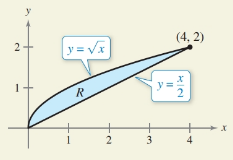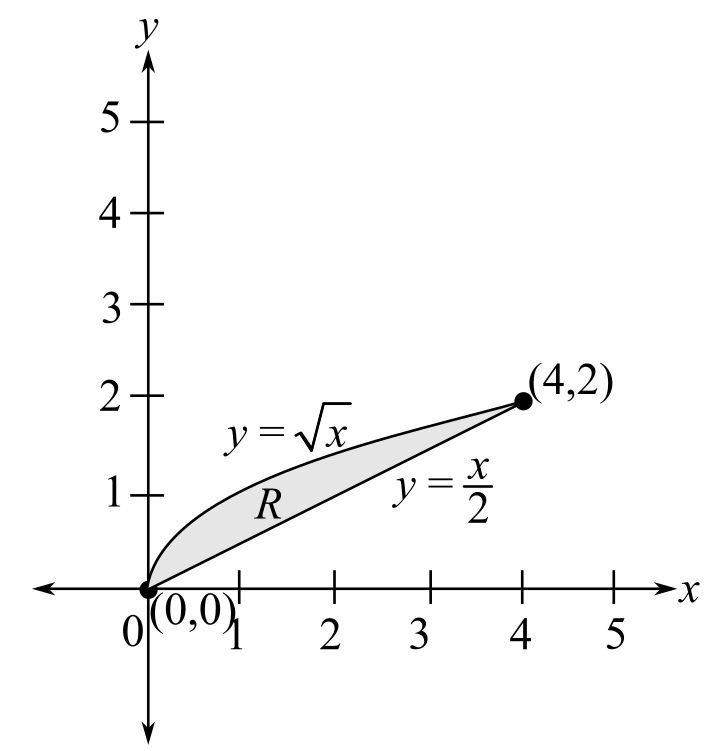Chapter 7.8, Problem 50E### Calculus: An Applied Approach (Min...

10th Edition
Ron Larson
ISBN: 9781305860919

#### Solutions

Chapter
Section### Calculus: An Applied Approach (Min...

10th Edition
Ron Larson
ISBN: 9781305860919
Textbook Problem
1 views

# HOW DO YOU SEE IT? Complete the double integrals so that each one represents the area of the region R (see figure).(a) A r e a = ∫ ∫ d x   d y (b) A r e a = ∫ ∫ d y   d x(a)

To determine

The complete double integral for the expression Area=dx dy such that it represents the area of the region R shown below in the figure as,Explanation

Given Information:

The provided expression is Area=dx dy and the graph of the region R is shown below in the figure as,

Consider the expression Area=dx dy

By observation of the provided graph, it is observed that the region R is bounded on the left by the equation y=x and on the right by the line y=x2.

So, on solving for y in the equation y=x the bounds for x would be y2x2y, which implies that it is the inner limit of integration

(b)

To determine

The complete double integral for the expression Area= dydx such that it represents the area of the region R shown below in the figure as,### Still sussing out bartleby?

Check out a sample textbook solution.

See a sample solution

#### The Solution to Your Study Problems

Bartleby provides explanations to thousands of textbook problems written by our experts, many with advanced degrees!

Get Started

#### Evaluate the limit, if it exists. limu24u+13u2

Single Variable Calculus: Early Transcendentals, Volume I

#### In Exercises 4562, find the values of x that satisfy the inequality (inequalities). 50. 0 x + 2 4

Applied Calculus for the Managerial, Life, and Social Sciences: A Brief Approach

#### Evaluate in problems 1-4. Write all the answers without using exponents. 3. (a) ...

Mathematical Applications for the Management, Life, and Social Sciences

#### Sometimes, Always, or Never: limxaf(x)g(x)=limxaf(x)limxag(x).

Study Guide for Stewart's Single Variable Calculus: Early Transcendentals, 8th

#### The area of the parallelogram at the right is: 26

Study Guide for Stewart's Multivariable Calculus, 8th